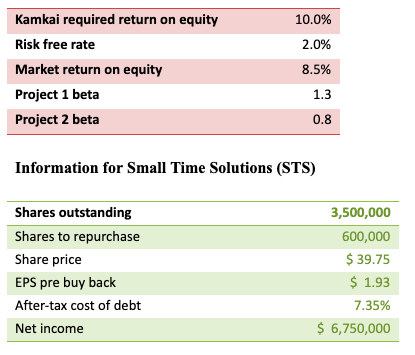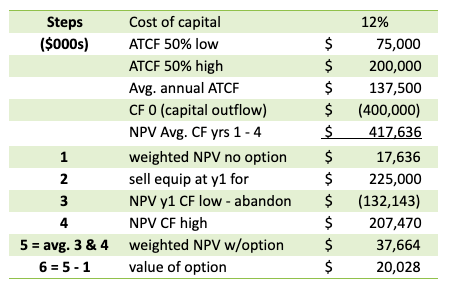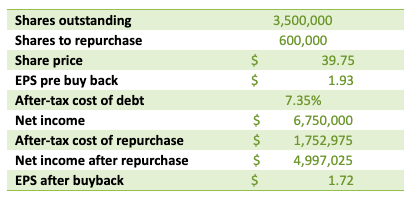# CFA Level II Exam - Sample Item Set Questions

In the CFA Level II Exam, the questions are asked in the form of item sets. First a short case or vignette is presented to the candidate followed by 6 multiple choice questions that need to be answered based on the information presented in the vignette. CFA Institute refers to these questions as Item Sets. Unlike independent multiple choice questions in CFA Level 1 exams, these item set questions have an added layer of difficulty since the examiner can test your knowledge on various concepts with fewer questions. There is also the risk that if you don't understand one part of the vignette you may be stuck with the entire 6 question item set.

The best way to be prepared is to attempt as many practice questions in the form of item sets as possible. Provided below is a sample item set taken from the Financial Reporting and Analysis section.

Below is one full item set for CFA Level II exam.

### Sample Item Set for CFA Level II

New App Corporation (NAC) is considering developing a new hardware component for a new handheld mobile device that is expected to hit the retail market next year.  There are significant capital costs associated with the new hardware project. If response to the new mobile device is strong, this could be a lucrative project for NAC.  Below are the details:

+ Low after tax cash flow/year scenario = $75 million; 50% probability + High after tax cash flow/year scenario =$200 million; 50% probability

+ Upfront capital spend = $400 million + Cost of capital = 12% + NAC can abandon the project after year 1 and sell the equipment for$225 million

+ Project term = 4 years

Kamkai is a competitor to NAC in the mobile device technology space.  Like many players in this industry, Kamkai has no debt. Below is info about Kamkai and two projects that it is considering:1. What is the value of NAC’s abandonment option at the end of year 1?

1. $17.6 million 2.$20.0 million
3. $37.7 million 2. Which of the following would not be considered a management flaw in making capital budget decisions? 1. Use of a standard corporate modeling template to value projects. 2. Emphasizing GAAP accounting implications when making a capital budget decision. 3. Utilizing NPV to decide between mutually exclusive projects. 3. If Kamkai uses its required return on equity to discount cash flows for projects 1 and 2, what are the likely effects on the NPV calculation? 1. Project 1is overvalued and project 2 is undervalued. 2. Project 1 is undervalued and project 2 is overvalued. 3. Both projects will be overvalued. 4. An analyst is determining the value of a capital budgeting project by netting cash flows against the economic depreciation of the assets to be purchased. This best describes which alternative to standard NPV analysis. 1. Economic income 2. Economic profit 3. Residual income 5. Which of the following is an example of an agency cost? 1. Providing a CEO with stock options for hitting earnings per share targets. 2. The cost associated with generating an annual report to shareholders. 3. The cost of establishing non-compete agreements for a company’s sales executives. 6. The notion that managers may use the least visible form of financing (such as retained earnings) before using more visible forms of financing (such as issuing debt or equity) to finance business pursuits best describes: 1. Static tradeoff theory 2. Pecking order theory 3. Modigliani-Miller theory of capital structure with taxes 7. Countries with weak legal systems are expected to have companies with: 1. Lower financial leverage and shorter average debt maturities. 2. Higher financial leverage and longer average debt maturities. 3. Higher financial leverage and shorter average debt maturities. 8. If STS proceeds with a share repurchase by issuing debt, what will be the earnings per share impact to shareholders? 1. Dilutive 2. Accretive 3. EPS neutral 9. Which of the following best describes the US dividend taxation policy? 1. Double taxation system 2. Split rate system 3. Imputation system ### Answers #1) Correct = B The table below walks through the calculation of the value of the option to abandon.#2) Correct = C Utilizing IRR to decide between mutually exclusive projects would risk making an error; NPV is the preferred decision rule. Choices A and B both represent common capital budgeting pitfalls, as standard templates may not be appropriate for all projects and a focus on accounting results could lead to less than optimal financial outcomes. #3) Correct = A First the analyst must calculate Kamkai’s beta, which is 1.2. Because this is lower than the risk of project 1, Kamkai would overvalue project 1 (i.e., the discount rate should be increased for project 1). Alternatively, project 2 is less risky than the company overall, so project 2 would be undervalued (i.e., the discount rate should be decreased for project 2). #4) Correct = A While all three are closely related, this situation best describes economic income. Economic income = after tax op cash flows – (begin market value of asset – end mv of asset) Economic profit = EBIT*(1- tax) – WACC*(book value of assets) Residual income = net income – (required return on equity * beginning book value of equity) #5) Correct = A Choice B is an example of a monitoring cost; Choice C is an example of a bonding cost. Both of these are net agency costs of equity. Choice A may be an incentive to manipulate accounting results and thus provide gain to the CEO at the expense of shareholders, exacerbating a conflict of interest rather than reducing it. #6) Correct = B This question is a description of the pecking order theory, whereby management seeks to avoid sending signals to the public, who may interpret issuing debt or equity in a variety of ways. The static tradeoff theory posits that the optimal capital structure is achieved when the costs of financial distress equal the tax shield on interest payments. M&M theory of capital structure with taxes is a theory that argues for 100% debt financing, as it assumes no financial distress costs so the company must maximize value from the interest tax shield. #7) Correct = C Empirical studies have shown that companies in countries with weak legal systems tend to have higher than average financial leverage with greater use of short term, rather than long term financing. #8) Correct = A First step is to calculate the after tax cost of the buy back, which is the number of shares multiplied by the price per share multiplied by the after tax cost of debt. Then subtract this value from net income. Take that result and divide by the new number of shares outstanding. As the table below shows, EPS declines from$1.93 to \$1.72.  A short cut can be done by checking to see if the after tax cost of borrowing exceeds the earnings yield.#9) Correct = A

In the US corporate income is taxed whether distributed to shareholders or not; then dividends are again taxed for shareholders when shareholders receive a cash dividend.

Under a split rate system, retained earnings are taxed at a different rate than earnings distributed as dividends to shareholders (typically distributions are taxed at a lower rate).

Under an imputation system, companies pay corporate taxes and dividend receiving shareholders can then deduct a portion of the taxes paid by the company from their own tax bill.

#### Post Resources

Practice Questions for CFA Level 2 Exam
Member-only